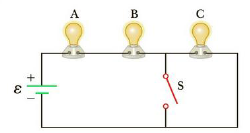Chapter 18, Problem 53AP

Chapter
Section
Textbook Problem

A circuit consists of three identical lamps, each of resistance R, connected to a battery as in Figure P18.53. (a) Calculate an expression for the equivalent resistance of the circuit when the switch is open. Repeat the calculation when the switch is closed. (b) Write an expression for the power supplied by the battery when the switch is open. Repeat the calculation when the switch is closed. (c) Using the results already obtained, explain what happens to the brightness of the lamps when the switch is closed.Figure P18.53

To determine
The value of R.

Explanation

When the switch is closed, the circuit can be reduced as,

When the circuit is open, the circuit can be reduced as,

The resistance between the points a and b drops to one-half of its original value when the switch is closed. Therefore,

R+18Ω=12(R+</

Still sussing out bartleby?

Check out a sample textbook solution.

See a sample solution

The Solution to Your Study Problems

Bartleby provides explanations to thousands of textbook problems written by our experts, many with advanced degrees!

Get Started

What is a standard unit?

An Introduction to Physical Science

Why is the Moon red during a total lunar eclipse?

Horizons: Exploring the Universe (MindTap Course List)

2. In healthy men, the hematocrit is about

Cardiopulmonary Anatomy & Physiology

What are the types of ocean waves, arranged by disturbing force and wavelength?

Oceanography: An Invitation To Marine Science, Loose-leaf Versin